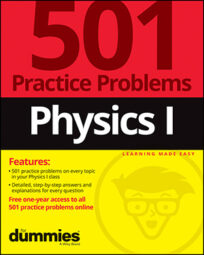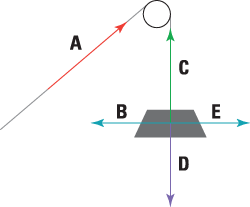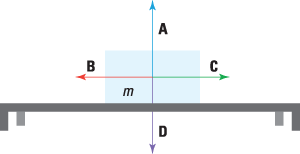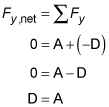##### Physics I: 501 Practice Problems For Dummies (+ Free Online Practice)In physics, free-body diagrams help you understand how Newton's laws of motion describe how objects move when forces are applied to them. Here are some practice questions that you can try.

## Practice questions

Use the following force diagram of a mass hanging from a pulley by a massless rope to answer Questions 1 to 3. Letters on the diagram signify vectors.1. Which vector represents the gravitational force Earth exerts on the mass?

2. Which vector(s) represent the force of tension?

3. Which two vectors always have equal magnitudes?

Use the following force diagram, showing a box of mass m sitting on a tabletop, to answer Questions 4 and 5. Letters on the diagram signify vectors.4. Which vector represents the normal force exerted on the box?

5. Assuming the table completely supports the box's weight, what equality must be true in terms of A, B, C, and D — the magnitudes of the vectors displayed?

The following are the answers to the practice questions:

1. D

The force of gravity between Earth and an object always points straight down in a force diagram.

2. A and C

Regardless of direction, tension forces always connect one object — upon which the force is exerted — to a second object that exerts the force. Anytime you see a taut rope in a physics question, tension forces exist along the rope. (In the given diagram, the direction of A indicates that the rope happens to be pulling up a second, unseen object with the help of the pulley.)

3. A and C

If the mass doesn't accelerate vertically, then C and D must be equal; likewise, if the mass doesn't accelerate horizontally, then B and E must be equal. However, neither of those situations is stipulated by any information you've been given. The only thing you know for sure is that the magnitudes of the two tension forces — A and C — must be equal, because they are on the same rope. They point in opposite directions to satisfy Newton's third law of equal-and-opposite forces (or else the rope would rip itself apart).

4. A

The normal force points perpendicularly away from a surface, starting from the point of contact between the object in question and the surface itself.

5. A = D

Completely supported by the table, the block never accelerates downward (or upward), so the net force on the block in the vertical direction must equal 0:Note that there is a negative sign on the magnitude of D because that vector is pointing down (the negative direction, conventionally). The magnitudes of A and D are equal, but their directions are opposite.